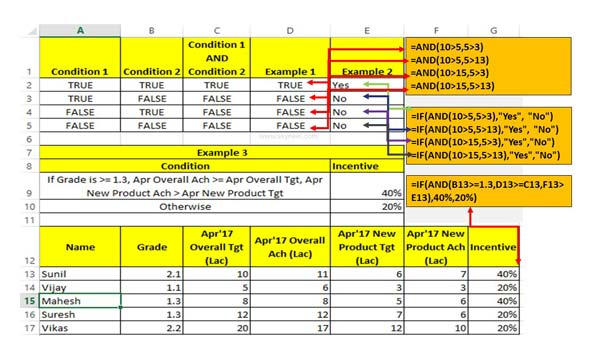# How to using logical AND function in Excel

AND function in Excel allows you to check multiple conditions and return TRUE if all given conditions evaluated to True.

If you want to compare different cells data or values then you must have to know how to using logical functions in Excel. Logical AND function in Excel is one of the most important and useful function of excel. If you have more than one criteria in that case you have to use logical AND function in Excel sheet to get the correct result.

The AND function is an most popular and important logical function. If you have more than one conditions and want to get correct result which match the given conditions then you have to use AND function in Excel. Normally AND function check all the given conditions and return the result TRUE if all the given conditions evaluated to True. If any one or more than one conditions does not match then this function evaluated to FALSE.

### AND Function in Excel

AND is an popular logical function which returns TRUE, if all given values or arguments are TRUE. This function returns FALSE if any single or multiple arguments evaluated FALSE.

### Syntax AND function

AND(logical1,logical2, …)

Logical1, logical2, logical3, … are 1 to 255 conditions.

If you are using AND along with IF function in Excel then this function returns TRUE when both condition evaluated True. If anyone given condition does not match the criteria then this function generate False result. Most of the person have to use AND function in Excel along with IF function to solve complex calculations.

## How to use logical AND function in Excel

If you have more than one criteria or condition then you must have to know how AND function works properly. Here we will discuss how to use logical AND function to solve complex task.

Example 1:

You can use AND function separately on different situation and check what effect is shown in the resulted area.

Example 2:

You can also use AND function with the help of IF function in Excel to view the effect on more than one conditions.

Example 3:

If you have complex criteria in which you have to use more than two conditions. In that situation you must have to use IF function along with AND function to get the correct result.I hope after reading this article you can easily use logical AND function. This guide helpful for all those Excel users who want to become an expert in Excel. If you have any suggestion regarding this guide then please write us your views in the comment box. Thanks to all.